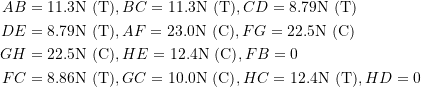### Problems: Chapter 6

1) Determine the member forces of the trusses shown.

Truss 1: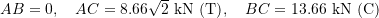Truss 2: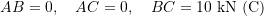2) Determine the member forces of the trusses shown.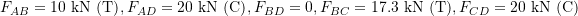3) Determine the member forces of the truss shown. Hint: some members are zero-force members.

Zero force members: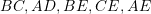and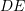. Non-zero force members: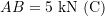and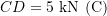.

4) If each member of the truss shown can resist a maximum member force of 5kN, determine the highest magnitude of the point load F that can be applied to the truss. Hint: some members are zero-force members.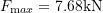.